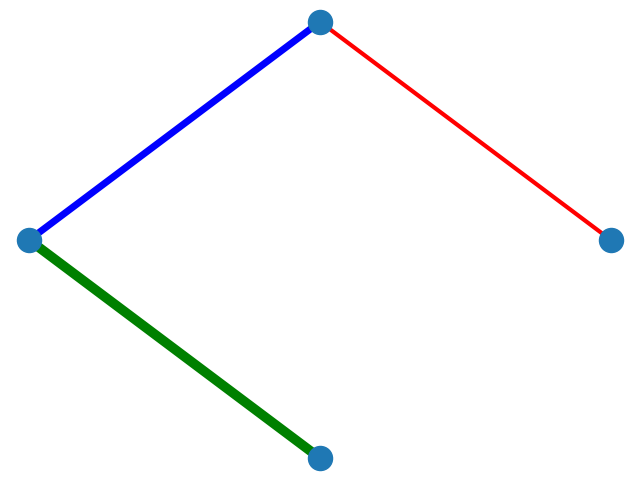# Drawing basics

## 1. How to draw directed graphs using NetworkX in Python?​

By using the base class for directed graphs, DiGraph(), we are able to draw a directed graph with arrows to indicate the direction of edges.

``import networkx as nximport matplotlib.pyplot as pltG = nx.DiGraph()G.add_edges_from(    [('A', 'B'), ('A', 'C'), ('D', 'B'), ('E', 'C'), ('E', 'F'),     ('B', 'H'), ('B', 'G'), ('B', 'F'), ('C', 'G')])nx.draw(G)plt.show()``

The output is:## 2. How to draw a NetworkX graph with labels?​

If you want the node labels to be visible in your drawing, just add `with_labels=True` to the `nx.draw` call.

``import networkx as nximport matplotlib.pyplot as pltG=nx.Graph()G.add_edge("Node1", "Node2")nx.draw(G, with_labels = True)plt.show()``

The output is:## 3. How to change the color and width of edges in NetworkX graphs according to edge attributes?​

Dictionaries are the underlying data structure used for NetworkX graphs, and as of Python 3.7+ they maintain insertion order. You can use the `nx.get_edge_attributes()` function to retrieve edge attributes. For every run, we are guaranteed to have the same edge order.

``import networkx as nximport matplotlib.pyplot as pltG = nx.Graph()G.add_edge(1, 2, color='r' ,weight=3)G.add_edge(2, 3, color='b', weight=5)G.add_edge(3, 4, color='g', weight=7)pos = nx.circular_layout(G)colors = nx.get_edge_attributes(G,'color').values()weights = nx.get_edge_attributes(G,'weight').values()nx.draw(G, pos, edge_color=colors, width=list(weights))plt.show()``

The output is:## 4. How to color nodes in NetworkX graphs?​

You need to define a color map that assigns a color to each node. For example, if were to color the first 15 nodes of a graph in `orange`, and the rest in `blue`, then the code would be:

``import networkx as nximport matplotlib.pyplot as pltG = nx.karate_club_graph()color_map = []for node in G:    if node < 15:        color_map.append('orange')    else:         color_map.append('blue')      nx.draw(G, node_color=color_map, with_labels=True)plt.show()``

The output is:## 5. How to color nodes in NetworkX graphs according to their attributes?​

You need to define a color map that assigns a color to each node.

``import networkx as nximport matplotlib.pyplot as pltG = nx.Graph()G.add_node('A', color='red')G.add_node('B', color='green')G.add_node('C', color='blue')G.add_edges_from(    [('A', 'B'), ('A', 'C'), ('B', 'C')])colors = [node['color'] for node in G.nodes(data=True)]nx.draw(G, node_color=colors, with_labels=True, font_color='white')plt.show()``

The output is: